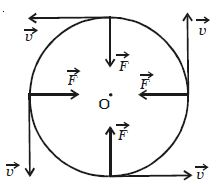# Explain Centripetal Force

According to Newton’s first law of motion, a body possesses the property called directional inertia; the inability of the body to change its direction. This means that without the application of an external force, the direction of motion can not be changed. Thus when a body is moving along a circular path, some force must be acting upon it, which continuously changes the body from its straight-line path (Figure).

It makes clear that the applied force should have no component in the direction of the motion of the body or the force must act at every point perpendicular to the direction of motion of the body. This force, therefore, must act along the radius and should be directed towards the centre.Hence for circular motion, a constant force should act on the body, along the radius towards the centre and perpendicular to the velocity of the body. This force is known as centripetal force.

If m is the mass of the body. then the magnitude of the centripetal force is given by

F = mass x centripetal acceleration

= m (v2/r) = mv2/r = m (rω2)